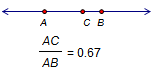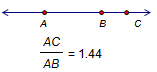# RatioSelection prerequisites: Two segments or three collinear points

Collinear points are points that lie on the same straight line.

This Measure menu command measures the ratio of two lengths or distances.

If two segments are selected, this command measures the ratio of the length of the first selected segment to the length of the second.

If three collinear points A, B, and C are selected in order, this command measures the ratio of the distance AC to the distance AB.

If points B and C are on the same side of A, the ratio is positive; if B and C are on opposite sides of A, the ratio is negative.

Another way to think of the ratio defined by three collinear points is to think of a number line with its origin at point A and its unit point at B. The position of point C on this number line determines the value of the measurement.C is between A and B, so the ratio is between 0 and 1.C is beyond B, so the ratio is greater than 1.C is beyond A, so the ratio is less than 0.

If point C is constructed on straight object AB, the ratio measurement is the same as the value of point C on its path. In this case it’s easier to select only point C and choose